# High School Chemistry : Help with Properties of Gases

## Example Questions

### Example Question #1 : Gases And Gas Laws

Which of the following gases will diffuse the quickest through a small hole?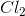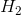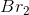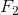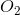Explanation:

Gases with high higher molecular weights are going to move at slower velocities. At a given temperature, all gases have the same average kinetic energy. For this to remain true, larger molecules must move slower since they have greater masses.

The gas to diffuse quickest will have the smallest molecular weight. In this case, that gas is hydrogen.

### Example Question #2 : Gases And Gas Laws

Which of the following is not a characteristic of gases?

High densities

Kinetic energy is dependent on temperature

Can diffuse in containers

Can effuse through small openings

High densities

Explanation:

Gases are able to effuse though small pinhole openings, and diffuse into empty spaces from high to low concentrations. The kinetic energy of gas molecules is dependent on temperature. Higher temperatures cause in increase in the kinetic energy of the particles.

Gases have very low densities. Density is a measure of mass per unit volume. Since the gas molecules are spread out over a much larger distance compared to liquids and solids, their densities are very low.

### Example Question #1 : Gases And Gas Laws

A container filled with fluorine gas and neon gas and has a total pressure of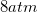. There are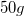of fluorine gas in the container, and the fluorine gas exerts a pressure of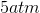.

Based on this, what is the mass of neon in the container?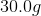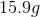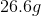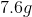Explanation:

The partial pressures of each gas are not dependent on their masses, but the total number of moles of gas in the container. Since we know how much pressure the fluorine gas exerts on the container, we can solve for the molar fraction of fluorine gas in the container.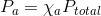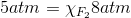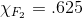In other words, 62.5% of the gas in the container is fluorine gas. Knowing this, we can solve for how many moles of neon gas are in the container.

50 grams of fluorine gas is equal to 1.32 moles of fluorine gas. If this molar amount accounts for 62.5% of the gas in the container, we can solve for the total number of moles in the container: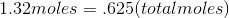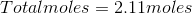Since there are only two gases in the container, we can solve for the number of moles of neon gas in the container.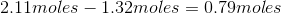Since there are .79 moles of neon gas, we can multiply by the molar mass and find the toal mass of the neon gas in the container: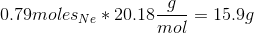### Example Question #4 : Gases And Gas Laws

Which of the following is a physical property of gases?

Compressibility

Low density

Fluidity

Explanation:

There are six primary properties of gases: expansion, fluidity, low density, compressibility, diffusion, and effusion.

Expansion suggests that gases have no defined shape and will expand to fill a given space, without significant intermolecular interaction. Fluidity is a property of both gases and liquids and describes the relatively low attraction between particles. This allows the gas molecules to move past one another, creating the "fluid" nature of the gas. Low density of gases is linked to gas expansion. Gases will expand to the greatest extent possible, resulting in low mass per unit volume ratios. Compressibility is also linked to expansion and the indefinite shape of the gas, essentially suggesting that the distance between particles can be reduced if pressure is increased. Diffusion and effusion are both linked to the movement of gases. Diffusion means that gases can spread out and mix within a given space, while effusion means that gases can pass through a small opening at a given rate.

Some of these properties are unique to gases, while others are shared between gases and liquids. Gases have virtually no physical properties in common with solids.

### Example Question #5 : Gases And Gas Laws

Which of the following gas laws can be used to determine the total pressure of a mixture of gases?

The second law of thermodynamics

Boyle's law and the pressure-volume relationship

Gay-Lussac's law and the pressure-temperature relationship

Dalton's law of partial pressures

Dalton's law of partial pressures

Explanation:

Each gas in a mixture of gases exerts its own pressure independently of the other gases present; therefore the pressure of each gas within a mixture is called the partial pressure of the gas.

Dalton's law of partial pressures states that the total pressure of a mixture of gases is equal to the sum of the partial pressures of the component gases. This can be expressed mathematically as follows: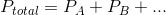Boyle's law and Gay-Lussac's law can help determine pressure in varying volumes and temperatures, respectively, but can only be useful with regard to the total pressure of the system. The second law of thermodynamics is not related to gas properties, and states that the entropy of the universe is constantly increasing.

### Example Question #1 : Gases And Gas Laws

Assume air contains 21% oxygen and 79% nitrogen.

If air is compressed to 5.5atm, what is the partial pressure of the oxygen?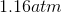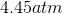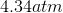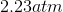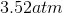Explanation:

Use Dalton's law of partial pressure: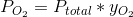Where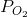is the partial pressure of oxygen and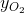is the mole fraction of oxygen. Plug in known values and solve.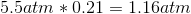### Example Question #1 : Gases And Gas Laws

Mass density is the grams of gas per volume, while number density is the number of molecules per volume.

Which of the following has the highest number density, if each gas occupies the same volume?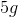of oxygen gasof methane gas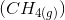of argon gas

All of these have the same number densityof hydrogen gasof hydrogen gas

Explanation:

Divide each component by their molecular weight to obtain the number of moles. The hydrogen gas has the most moles that occupy the same volume. Remember that of the answer choices, hydrogen, and oxygen are both diatomic gasses, which needs to be taken into account when calculating the number of moles. For simplicity, let's assume we have 1L of each gas.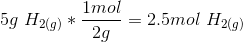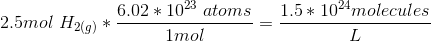As an example, let's calculate the number density for oxygen to show that it is indeed less than that for hydrogen.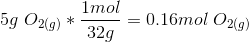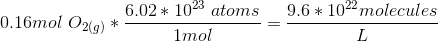### All High School Chemistry Resources